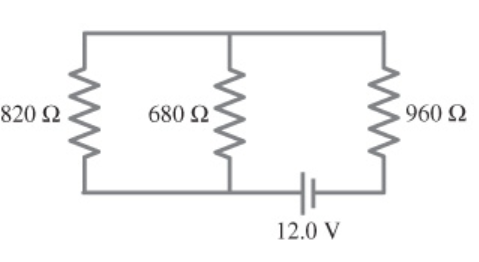# Problem: (a) Determine the equivalent resistance of the circuit shown in the figure. Express your answer to three significant figures and include the appropriate units.(b) Determine the voltage across 820 Ω resistor. Express your answer to two significant figures and include the appropriate units.(c) Determine the voltage across 960 Ω resistor. Express your answer to two significant figures and include the appropriate units.(d) Determine the voltage across 680 Ω resistor. Express your answer to two significant figures and include the appropriate units.￼

###### FREE Expert Solution

Equivalent resistance for series resistors:

$\overline{){{\mathbf{R}}}_{\mathbf{e}\mathbf{q}}{\mathbf{=}}{{\mathbf{R}}}_{{\mathbf{1}}}{\mathbf{+}}{{\mathbf{R}}}_{{\mathbf{2}}}}$

Equivalent resistance for parallel resistors:

$\overline{)\frac{\mathbf{1}}{{\mathbf{R}}_{\mathbf{eq}}}{\mathbf{=}}\frac{\mathbf{1}}{{\mathbf{R}}_{\mathbf{1}}}{\mathbf{+}}\frac{\mathbf{1}}{{\mathbf{R}}_{\mathbf{2}}}}$

(a) The 820Ω and the 680Ω resistor are in parallel.

R820,680 = (1/820 + 1/680)-1 = 371.7 Ω

83% (162 ratings)###### Problem Details

(a) Determine the equivalent resistance of the circuit shown in the figure. Express your answer to three significant figures and include the appropriate units.
(b) Determine the voltage across 820 Ω resistor. Express your answer to two significant figures and include the appropriate units.
(c) Determine the voltage across 960 Ω resistor. Express your answer to two significant figures and include the appropriate units.
(d) Determine the voltage across 680 Ω resistor. Express your answer to two significant figures and include the appropriate units.